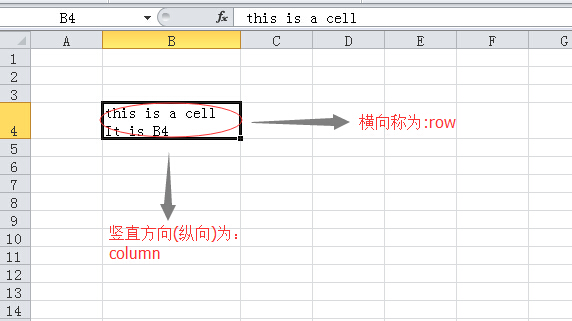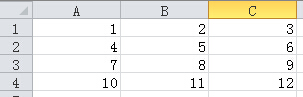AI教程网 - 未来以来，拥抱AI；新手入门，从AI教程网开始......

# 零基础学Python:电子表格

118℃

## openpyl

openpyl模块是解决Microsoft Excel 2007/2010之类版本中扩展名是Excel 2010 xlsx/xlsm/xltx/xltm的文件的读写的第三方库。（差点上不来气，这句话太长了。）

### 安装

``````\$ sudo pip install openpyxl
``````

``````Successfully installed openpyxl jdcal
Cleaning up...
``````

### workbook和sheet

``````>>> from openpyxl import Workbook
``````

``````>>> wb = Workbook()
``````

``````>>> ws = wb.active
``````

``````>>> ws1 = wb.create_sheet()
``````

``````>>> ws2 = wb.create_sheet(1)
``````

``````>>> ws.title = "python"
``````

ws所引用的sheet对象名字就是”python”了。

``````>>> ws01 = wb['python']    #sheet和工作簿的关系，类似键值对的关系
>>> ws is ws01
True
``````

``````>>> ws02 = wb.get_sheet_by_name("python")    #这个方法名字也太直接了，方法的参数就是sheet名字
>>> ws is ws02
True
``````

``````>>> print wb.get_sheet_names()
['python', 'Sheet2', 'Sheet1']
``````

Sheet2这个sheet之所以排在了第二位，是因为在建立的时候，用了一个加塞的方法。这跟Excel中差不多少，如果sheet命名了，就按照那个名字显示，否则就默认为名字是”Sheet1″形状的（注意，第一个字母大写）。

``````>>> for sh in wb:
...     print sh.title
...
python
Sheet2
Sheet1
``````

### cell``````b4 = ws['B4']
``````

``````>>> ws['B4'] = 4444
``````

``````>>> b4.value
4444
``````

``````>>> a1 = ws.cell("A1")
``````

``````>>> a2 = ws.cell(row = 2, column = 1)
``````

|A1|B1|C1|
|A2|B2|C2|
|A3|B3|C3|

``````>>> cells = ws["A1":"C3"]
``````

``````>>> tuple(ws.iter_rows("A1:C3"))
((<Cell python.A1>, <Cell python.B1>, <Cell python.C1>),
(<Cell python.A2>, <Cell python.B2>, <Cell python.C2>),
(<Cell python.A3>, <Cell python.B3>, <Cell python.C3>))
``````

``````>>> for row in ws.iter_rows("A1:C3"):
...     for cell in row:
...         print cell
...
<Cell python.A1>
<Cell python.B1>
<Cell python.C1>
<Cell python.A2>
<Cell python.B2>
<Cell python.C2>
<Cell python.A3>
<Cell python.B3>
<Cell python.C3>
``````

``````>>> ws.rows
((<Cell python.A1>, <Cell python.B1>, <Cell python.C1>),
(<Cell python.A2>, <Cell python.B2>, <Cell python.C2>),
(<Cell python.A3>, <Cell python.B3>, <Cell python.C3>),
(<Cell python.A4>, <Cell python.B4>, <Cell python.C4>))
``````

``````>>> ws.columns
((<Cell python.A1>, <Cell python.A2>, <Cell python.A3>, <Cell python.A4>),
(<Cell python.B1>, <Cell python.B2>, <Cell python.B3>, <Cell python.B4>),
(<Cell python.C1>, <Cell python.C2>, <Cell python.C3>, <Cell python.C4>))
``````

``````>>> i = 1
>>> for cell in ws.rows:
...     cell.value = i
...     i += 1
``````

Traceback (most recent call last):
File ““, line 2, in
AttributeError: ‘tuple’ object has no attribute ‘value’

``````>>> for row in ws.rows:
...     for cell in row:
...         cell.value = i
...         i += 1
...
``````

``````>>> for col in ws.columns:
...     for cell in col:
...         print cell.value
...
1
4
7
10
2
5
8
11
3
6
9
12
``````

### 保存

``````>>> wb.save("23401.xlsx")
``````### 读取已有文件

``````>>> from openpyxl import load_workbook
>>> print wb2.get_sheet_names()
['python', 'Sheet2', 'Sheet1']
>>> ws_wb2 = wb2["python"]
>>> for row in ws_wb2.rows:
...     for cell in row:
...         print cell.value
...
1
2
3
4
5
6
7
8
9
10
11
12
``````

## 其它第三方库

• xlrd：网络文件：https://secure.simplistix.co.uk/svn/xlrd/trunk/xlrd/doc/xlrd.html?p=4966